Mobile QR Code1. (Department of Electronics and Communication, The Oxford College of Engineering, Bangalore-560068, India)
2. (Department of Electronics and Communication Engineering, SRM Institute of Science and Technology, Kattankulathur, Chennai,Tamil Nadu, India)
3. (Laboratory of Computational Modeling of Drugs, Higher Medical and Biological School, South Ural State University, Chelyabinsk-454080, Russia )

Image restoration, BALLORG, Low-rank Prior, Augmented Lagrangian, Penalty methods, Lagrangian multipliers, Derivative prior, Block sparsity, Ill posed optimization, Constrained optimization

## 1. Introduction

Recent advances in the commercial electronics industry include increases in display resolution for visual image-output devices. This means that older images and videos shot on lower-resolution cameras can be blurred when displayed on the latest high-resolution screens. To counter this compatibility problem, we need to use super resolution along with deblurring algorithms to provide a high-quality output on the screen . The deblurring is needed in this instance because of the fact that the super resolution algorithm always tends to introduce slight blurriness or smoothness in the image, which can be removed using a deblurring algorithm.

The severity of these blurs is not that heavy and does not require the complexity of a learning-based method.

Most times, the blurs can be modelled with uniform and Gaussian features. There can be other areas of image capture and processing where we can encounter blur in images (mostly due to auto focal point error, handshake, camera movement, etc.).

As soon as the photons entering the cameras are converted to a voltage and quantified at a CMOS sensor, the only way to correct the image is with digital post-processing using digital imaging algorithms, such as the BALLORG method proposed here. Also, in many cases, the digital post-processing must happen before the image is viewed or transmitted, requiring real-time processing so that it can viewed seamlessly in high quality. As it is well established in the literature, we have deblurring algorithms that have ill-posed problems and seek an optimal solution for the ill-posed problem, which can be computationally expensive and require lengthier computation time.

The main focus of the majority of deblurring and digital reconstruction techniques is computation speed. Iterative loops that increase with the image size are used in high-resolution pixel-based image and video processing, which enable the coupling of an extremely powerful CPU with imaging technology. The proposed deblurring algorithm BALLORG is intended to solve the following problems: (i) it can provide satisfactory results both subjectively and visually; (ii) it has less computational complexity; (iii) it is able to perform better than similar algorithms; and (iv) it has a novel design.

The rest of the paper is organized as follows. We briefly examine some related work that makes use of prior-based and sparse approximation techniques in Section 2. In Section 3, we introduce the augmented lagrangian, low-rank prior-based deblurring algorithm BALLORG. In Section 4, experiments are discussed, and Section 5 shows the paper's conclusion.

## 2. Related Work

Image deblurring literature dates back to the 1960s with some of the first research aimed at determining the optimal ill-posed solution for deblurring as blind, semi-blind, and non-blind deconvolution with or without priors. The blind deconvolution can be used when a generalized approach can work well for blurred images with uniform anomality blur. Semi-blind and non-blind approaches  can be used for a variety of blurring phenomena, and each time, some information about the blur distribution is used as a prior in the deblurring algorithm.

Over the years, the deblurring algorithm has diversified rapidly to borrow algorithms from the area of computer science, artificial intelligence, and mathematics and is very versatile in its scope and application areas . Among the very prominent methods are wavelet-based methods , Wiener methods , edge-based methods, and compressive sensing methods . Researchers heavily depend on the use of priors in a deblurring algorithm, especially when the severity of the blurs is increased and the blur’s existence is unpredictable .

Now, we have an unknown blur variable with increasingly more unknown parameters depending on the priors, making it more manageable and predictable. Thus, the prior-based methods convert blind deblurring into semi-blind and non-blind categories  and can be seen to be deployed in the latest literature. The priors that have been used successfully include edge-based priors, gradient-based priors, wavelet-based priors, L norm priors , Gaussian mixture model priors , principal component analysis, singular value decomposition priors, etc. . Deblurring methods using compressive sensing have also proved efficient, and more variants methods in deblurring include wavelets, Bayesian, gaussian mixture, and evolutionary algorithms, etc.

Non-local central similarity (NCSR)  is one of the proposed algorithms based on non-local similarity in addition to dictionary learning. In this class of algorithms, we also have regularization-based approaches, low-rank prior, and sparse representation methods .The proposed algorithm BALLORG belongs to this category. Thus, in our experiments, we have used methods from this category for PSNR and FSIM comparison and evaluation of the proposed approach. The regularization method limits the solution space. Regularization algorithms based on total variation (TV)  offer high edge-maintaining properties. Quality wavelet-type filter-based approaches are used to extract multi-scale information. These proved to be of higher quality than the TV-based method.

Patch-based representation is more important than pixel-based representation. In image denoising, non-local means, imposing a regularization term, the K-SVD algorithm, the BM3D denoising algorithm, the low-rank minimization algorithm, and weighted nuclear norm minimization are often used approaches. Sparse representation is important in image processing. Processing is faster and easier in a sparse representation because only a few coefficients disclose the information we want. Such representations can be built by decomposing signals over elementary waveforms from a family known as a dictionary.

Since the first development of non-supervised algorithms and the popularity of dictionary approaches, there has been a significant amount of study on learning-based deblurring. These methods will be capable of dealing with both spatial and spatiotemporal blurring at a high rate. Many studies have attempted to solve the problem of image deblurring and super-resolution using deep learning. Convolutional neural networks (CNNs) , decision trees, large-scale CNNs, generative adversarial networks (GANs) , and other recent deep learning systems are examples. Algorithms like augmented Lagrangian , alternating multiplier direction methods (ADMMs) , Bregman iterations , proximal gradient method , and the Gauss Seidel method  showed tremendous improvement in the DL domain. BALLORG can be compared to TV regularization , Lagrangian deblurring methods , and alternating minimization methods classes of deblurring methods.

## 3. The Proposed Scheme

The suggested BALLORG algorithm's pipeline is depicted in Fig. 1. As evident from Fig. 1, it consists of the following interactive modules: (i) a low-rank prior module for sparse representation and faster optimization, (ii) a gradient prior for preserving sharp details, (iii) block-based processing to make use of local and global similarity details and to make it faster than pixel-level processing, (iv) augmented Lagrangian optimization with penalty weights for optimization, and (v) regularization parameter tuning, which leads to better separability of a sharp image and blur kernel.

The ill-posed problem is converted into constrained optimization using penalty based optimization technique called Augmented Lagrangian Method. The dimensionality reduction is done using Proximal gradient Approximation technique and Low rank Regularizer. Finally better image quality is achieved as result.

##### Fig. 1. The proposed BALLORG algorithm is depicted as a block diagram. The architecture shown above includes the augmented Lagrangian method, constrained optimization, proximal gradient low-rank prior, and gradient prior in horizontal, vertical, and diagonal directions.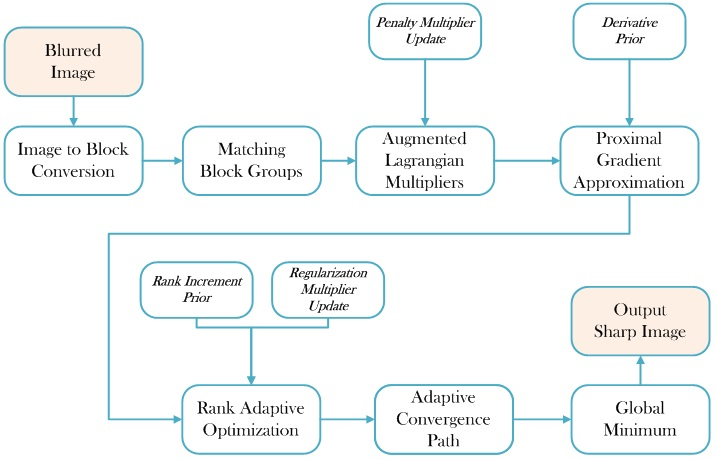### 3.1 Low-rank Optimization

As stated, the low-rank optimization problem is:

##### (1)
$\min _{x\in R^{MxN}}\parallel Hx-y\parallel +\lambda \parallel x^{*}\parallel$

where $\left\| Hx-y\right\|$ is the convex function, $\left\| x^{*}\right\|$ is a singularity in which the nuclear norm of$x$, which is derived. The rank matrix for optimization is x, and we plan to gradually raise its rank $x$ in $\lambda \left\| x^{*}\right\|$ iteratively. The initial rank of x is set to zero, and then the rank previous is gradually increased as shown below.

##### (2)
$\lambda _{k}rank\left\| x_{k}\right\| \geq \lambda _{k+1}rank\left\| x_{k+1}\right\| ,\,$

The rank prior $x^{*}$ is calculated in each iteration along with regularizer $\lambda _{k}$with some minor modification. As we increment the rank prior, the dependent rows and columns increase and help in achieving the global minimum. The low-rank prior can be incorporated in both the objective and penalty function as:

##### (3)
$\min _{x\in R^{MxN}}\parallel Hx-y\parallel +\lambda \parallel x^{*}\parallel s.t\,\,rank\left(x\right)<rank\left(x=x^{*}\right)$

The above equation can be with Frobenius norm with least-square approximation as

##### (4)
$\min _{x\in R^{MxN}}\parallel Hx^{*}-y\parallel _{F}^{2}+\lambda \parallel x^{*}\parallel$

In addition to assisting in principal component pursuit (PCP), the above low-rank prior approximation  can also overcome some noisy readings in the input image. By representing the convex hull as an augmented Lagrangian and providing the following information, constraint optimization can be reduced to unconstrained optimization:

##### (5)
$\min _{x\in R^{MxN}}\parallel Hx^{*}-y\parallel \,_{F}^{2}+\lambda \parallel x^{*}\parallel +\frac{\mu }{2}\parallel Hx^{*}-y+\lambda \parallel \nabla x\parallel \parallel \,_{F}^{2}$

where $\nabla x$ represents the gradient of the deblurred image in the X, Y, and Z directions, and $\mu$ is a parameter determined from the penalty regularizer variable $\lambda$. The scalar parameter values tend to keep the variable splitting very sparse and marginal and make it efficient for singular value selection:

##### (6)
$x^{*}=\lambda \left\| \sum _{i}\rho \upsilon \right\|$

where $\rho$and $\upsilon$are the right and left singular vectors, respectively. The fixed low-rank optimization in our approach is a matrix completion problem that uses Reimannian-structure trust-region coefficients for low-computation rank generation and rank incrementation by choosing the global minima over the desired local minima

##### (7)
$\min _{x\in \mathrm{\mathbb{R}}^{MxN}}\left\| H\sum _{i}\rho \upsilon -y\right\| _{F}^{2}+\lambda \left\| \sum _{i}\rho \upsilon \right\|$

The use of the sub-variable differential and gradient has been defined in a Reimannian structure and allows for faster computation in first-order optimality conditions given by:

##### (8)
$\nabla x_{k}+\lambda _{k}\nabla _{k}\left| \sum _{i}\rho \upsilon \right| =0$

The conditions above point the solution to the optimal global minimum without consideration of the closeness to the local minimum. This closeness can be made to synchronize using augmented Lagrangian penalty methods:

##### (9)
$\min _{x\in R^{MxN}}\parallel H\sum _{i}\rho \nu -y\parallel \,_{F}^{2}+\lambda \parallel \sum _{i}\rho \nu \parallel +~ \frac{\mu }{2}\parallel H\sum _{i}\rho \nu -y+\lambda \parallel \nabla x\left(D_{xyz}\right)\parallel \parallel \,_{F}^{2}$

where$D_{xyz}$represents the derivatives in horizontal, vertical, and diagonal directions. The low-rank Riemannian approach above takes all set of points inside a search set, as shown in Fig. 2. The search space’s initial values depend on the significant singular values in horizontal and vertical subspace, which can be moved as a block towards the direction of the global minimum.

However, the convergence of the rank does not stop the deblurring, which can still continue with the same rank until deblurring convergence is achieved. Fig. 2 shows the low-rank and low-range convergence direction with and without the derivative prior for two separate test pictures. It is clear that the low rank with the preceding derivative provides a much smoother convergence direction than using the low-rank prior alone. The same holds true for Gaussian, uniform, and motion blurred image

##### Fig. 2. The global minimum convergence using low-rank $\sum _{i}\rho \upsilon$ with derivative prior $D_{xyz}$: (a) Use of $D_{xyz}$ has some smoothing in the iterative convergence, whereas in (b), it does not seem to have too much influence. The graph illustrations have been plotted for two different images. }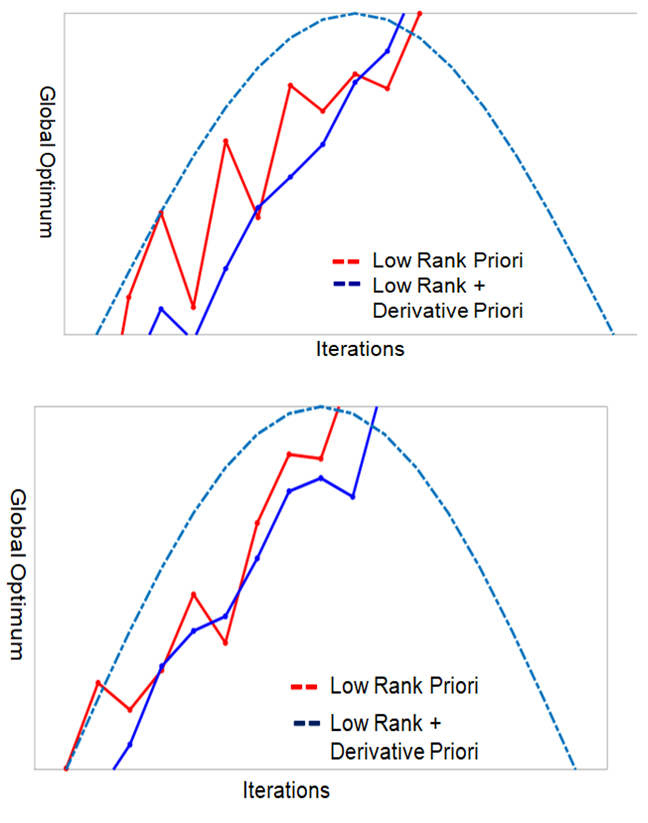### 3.2 Augmented Lagrangian Optimization with Low-rank and Derivative Prior

For remaining deblurring and convergence, the augmented Lagrangian optimization cost function employs the low-rank derivative prior [21-23]. The enhanced Lagrangian issue is modelled as:

##### (10)
$L_{c}\left(x,\lambda \right)=f\left(x\right)+\lambda h\left(x\right)+\frac{1}{2}c\left| h\left(x\right)\right| ^{2}$

The enhanced Lagrangian technique described above will now be combined with the low-rank derivative to keep it limited within the constrained set. Because of the following features, using an augmented Lagrangian simplifies convergence minimization even further. The function can be realized under first-order conditions since it is convex, differentiable, and has zero gradients. The function's boundaries are limited to $-\infty$ to $+\infty$. Using these conditions, we can now denote the prior-based augmented Lagrangian as:

##### (11)
$L_{c}\left(x,\lambda \right)=\min _{x\in \mathrm{\mathbb{R}}^{MxN}}\left\| H\sum _{i}\rho \upsilon -y\right\| _{F}^{2}+\lambda \left\| \sum _{i}\rho \upsilon \right\| +\frac{\mu }{2}\left\| H\sum _{i}\rho \upsilon -y+\lambda \left\| \nabla x\left(D_{xyz}\right)\right\| \right\| _{F}^{2}+\frac{1}{2}c\left\| \nabla x\left(D_{xyz}\right)\right\| ^{2}$

The augmented Lagrangian above has a penalty parameter and multipliers in ($\lambda ,\,\,c$), which must be computed along with other variables in each iteration that can be predefined for a rate of convergence as:

##### (12)
$\phi \left(\lambda ,c\right)=\rho ^{-1}\left| \lambda ,c\right| ^{p}+\frac{1}{2}\left| \lambda ,c\right| ^{2}$

where $\rho$(1,2) and $p>2$make the convergence super linear and overcome ill conditioning. The low-rank criteria that can guarantee convexity and convergence are given by:

##### (13)
$L_{c}\left(x,\lambda \right)=\lambda \left\| \sum _{i}\rho \upsilon \right\| +\frac{1}{2}c\left\| \nabla x\left(D_{xyz}\right)\right\| ^{2}$

By applying the one-sided equality constraints to the above, we have:

##### (14)
$L_{c}\left(x,\lambda \right)=\max \left(0,\lambda \left\| \sum _{i}\rho \upsilon \right\| \right)+\max \left(0,\frac{1}{2}c\left\| \nabla x\left(D_{xyz}\right)\right\| ^{2}\right)$

This leads to exact minimization for $\lambda ,c$:

##### (15)
$\nabla _{\lambda }\rho \left(\lambda ,c\right)=c+\nabla \phi \left(\lambda ,c\right)$

The criterion above computes ${\lambda}$ and c to give a unique point and attains minima.

## 4. Experimental Results

The suggested algorithm was compared to other current state-of-the-art methods in terms of performance and efficiency. The experimental comparison of algorithms was done with two categorical methods: prior-based and intelligence-based algorithms. Experiments were carried out on the standard datasets used in a comparative paper.

### 4.1 Performance Comparison with Prior-based Algorithms

In the deblurring category using full or semi-prior-based inputs, we used NCSR (Non-Locally Centralized Sparse Regularization), FISTA (Fast Iterative Shrinkage-Thresholding Algorithm), ASDS-REG (Adaptive Sparse Domain Regularization), IDD-B3D (Block Matching 3D Frames), and L0-SPAR (L0 Sparse Deblurring). Identical datasets and an experimental setup were used to do the performance comparison. For the test images, we used uniform and gaussian blur deformities with added random noise. Table 1 displays the results of the suggested ISNR approach for six different blur scenarios. Fig. 3 indicates that BALLORG converges faster and in less iteration compared to the prior-based techniques. For most of the images studied, the optimal convergence occurs after fewer than 50 repetitions.

##### Fig. 3. Low-rank versus iterations displaying the percentage of low-rank priors used for BALLORG deblurring on all the pictures in the dataset. The x-axis depicts the number of iterations, while the y-axis represents the intensity of the low-rank prior used throughout deblurring.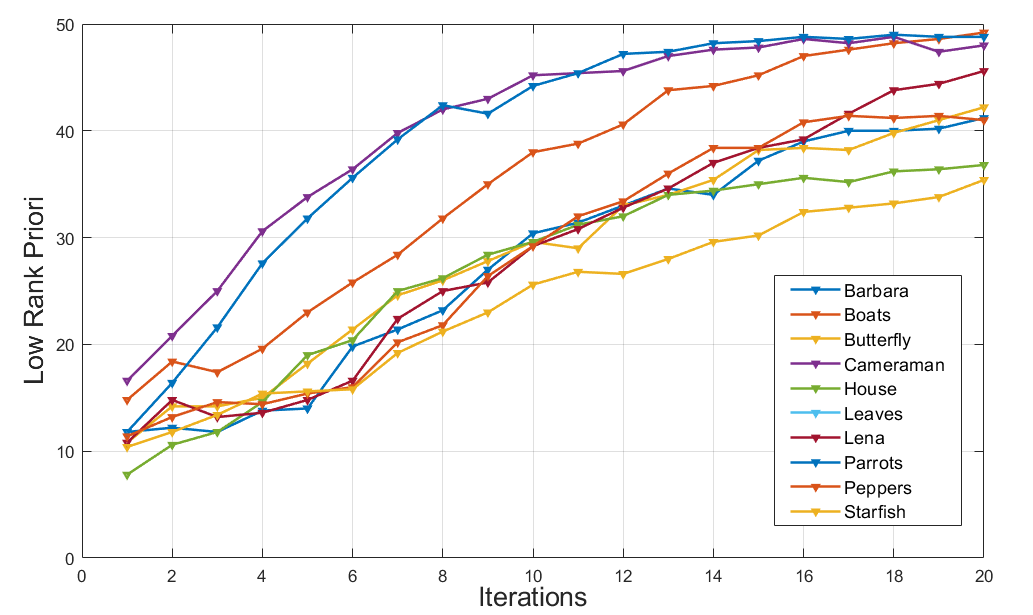##### Table 1. Comparison of the performance of existing techniques with BALLORG on uniform and Gaussian blur.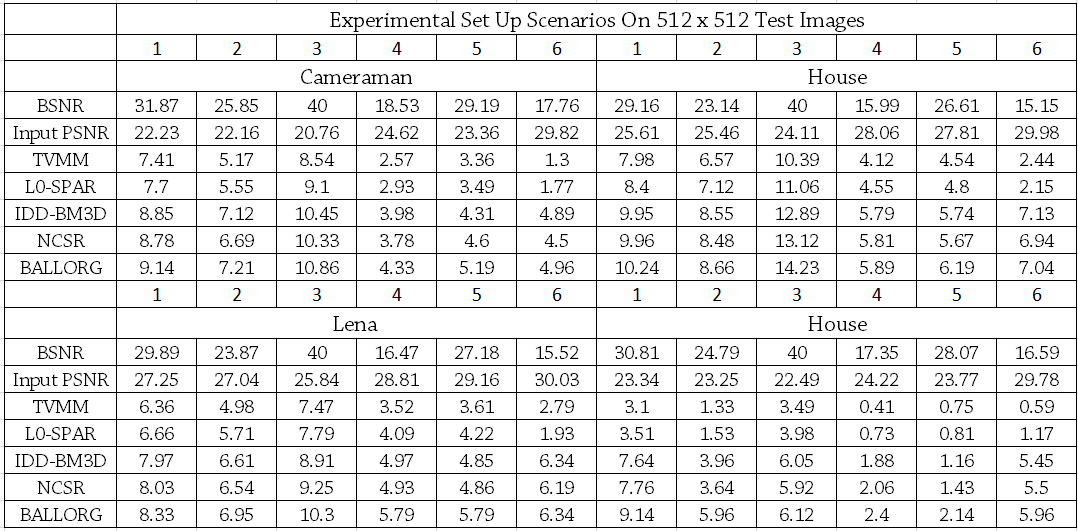### 4.2 Performance Comparison with Learning-based Methods

The proposed BALLORG method was also compared to other deep learning-based deblurring approaches proposed for scale recurrent deep image deblurring , neural blind motion deblurring, motion blur kernel deep learning, blind deblurring using conditional adversarial networks, and deep unrolling for image deblurring (DUBLID). All the algorithms described above have been evaluated for performance computation on the Berkeley Segmentation Dataset and the Benchmark Dataset. The techniques were tested for motion blur using 16 angles and interpolation, yielding 256 kernels.

Gaussian noise with a standard deviation of 0.01 was applied to 256 ${\times}$ 256 blurred photos. The images were then pipelined to the BALLORG method, and the peak signal-to-noise ratio (PSNR) and similarity index measure (SSIM) were computed. The results suggest that the proposed BALLORG method outperforms the deep learning-based deblurring algorithms given in Table 2.

##### Table 2. Comparison of proposed BALLORG method with deep learning methods on the same test images and kernels.
 Metrics Recurrent DL  Motion Kernel DL  Generative Adversarial Network  BALLORG PSNR 22.33 25.32 24.82 27.80 SSIM 0.76 0.83 0.80 0.88

### 4.3 Modular Algorithmic Performance Comparison

The proposed BALLORG method was disassembled into submodules to demonstrate the significant role of each module in the image restoration and sharpening. Fig. 4 depicts the results of the proposed BALLORG method on images from standard datasets. A comparison was done utilizing the present strategies. Fig. 4 displays the suggested algorithm's step-by-step outcomes. The figure's first column illustrates a uniformly blurred image with a kernel mask of 9X9 and noise deviation of 1.414. The gradient priors in horizontal, vertical, and diagonal dimensions are illustrated in the second column.

As can be seen in column 2 of Fig. 4, the use of these weighted gradient priors results in optimum edge preservation with each iteration, blur is progressively and iteratively reduced, and only the smoothest areas are used aggressively for deblurring. The low-weighted blue pixelated portions and larger-weighted yellow pixelated regions were plugged into the Lagrangian penalty characteristics. The regularized low rank given in the third column was used as a prior and updated with every iteration.

Table 3 compares the proposed BALLORG method with its similar modular counterpart, including the augmented Lagrangian method (ALM), augmented Lagrangian with low rank (ALLOR), and block-based augmented Lagrangian deblurring (BALM). The use of the low-rank and gradient Priors and the use of the block-based weighted degree calculated from the gradient priors improve performance metrics such as PSNR and SSIM.

##### Fig. 4. Experimental findings on test images. The first column contains the blurry test image, the second column contains the gradient previous weights used for deblurring, the third column contains the Lagrangian penalty term weighted by edge information, and the fourth column contains the low rank front edge map with regularization used to update and calculate the low rank to each iteration.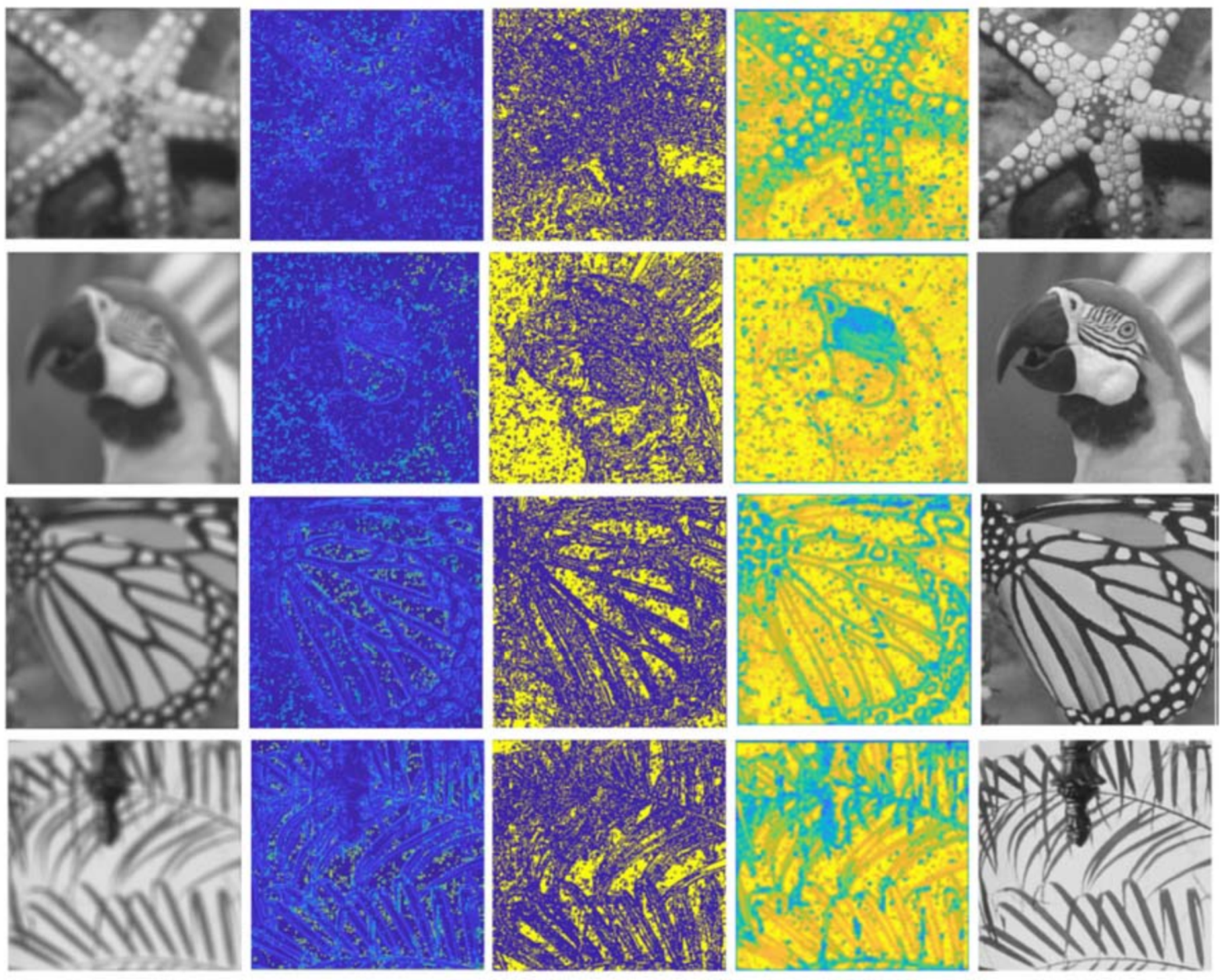##### Table 3. The suggested BALLORG method is compared to various relevant algorithms, such as augmented Lagrangian with low-rank prior (ALLOR), block augmented Lagrangian method (BALM), and augmented Lagrangian method (ALM).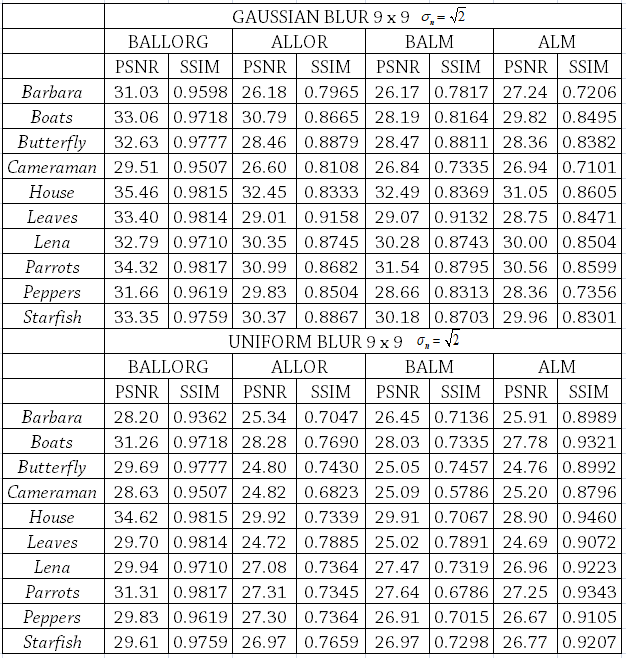### 4.4 Comparison of Blind Deblurring Techniques and BALLORG

The effectiveness of the suggested method is compared with current state-of-the-art algorithms in Table 4, including FISTA , L0-SPAR, IDD-B3D , ASDS-REG , and NCSR . In the studies, images with additive random variation and Gaussian and uniform blur were used. It is evident that BALLORG functions well for virtually any image with a constant parameter. The ISNR results of the proposed algorithm on six distinct blur scenarios demonstrate the usefulness of the suggested approach once more.

Fig. 5 demonstrates that BALLORG’s convergence occurs in far fewer iterations than that of existing approaches (1000 or more). For most of the studied photos, the optimum convergence occurs at 50 iterations and above, resulting in cases of overfitting or needless blurring. Because of the faster convergence, the suggested technique is suitable for real-time implementation.

The plot of PSNR vs. iterations proves that the suggested BALLORG deblurring can obtain optimum performance with a smaller number of iterations given in Fig. 5. Regarding rank priors vs. computation time, the use of low-rank priors has a negligible impact on computation time at each iteration.

##### Table 4. PSNR and SSIM comparison of BALLORG with several techniques: complete variational, sparse, 3D frame, adaptive sparse, and non-locally centralized sparse deblurring.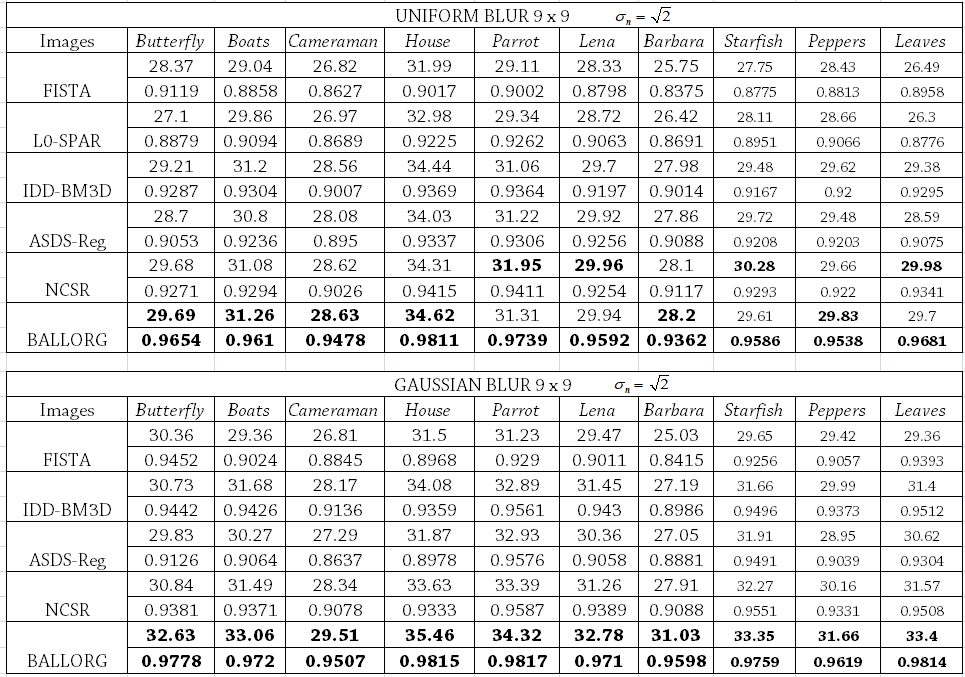## 5. Conclusion

We presented a novel BALLORG deblurring algorithm that seamlessly integrated augmented Lagrangian low-rank priors and gradient priors to produce improved efficiency output. The proposed approach is applicable to a wide range of blur severity and blur types, which include Gaussian and uniform blur. The least amount of iterations was required to reach minimization convergence, and the usage of rank prior had very little negative effect on the computing speed, making it faster than other approaches. The rank prior has only a little impact on the computational cost of the algorithm, but the fewer iterations make the process run faster.

BALLORG was compared to previous deblurring algorithms based on learning. Even though BALLORG has proven that low rank along with the augmented Lagrangian can be an ideal option for deblurring, parameter tuning was a little cumbersome and needed manual assistance to some extent. Through experimental simulations, the importance of each module in the pipeline and faster convergence were also shown. The future application of BALLORG includes the integration of this algorithm in devices running Android and mobile platforms. Future research will involve evaluating BALLORG on embedded systems and developing a system on chip for use in consumer technology products like phones, cameras, and televisions

### REFERENCES

1
H. Zheng, "A Survey on Single Image Deblurring," 2021 2nd International Conference on Computing and Data Science (CDS), 2021, pp. 448-452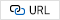2
M. El Helou and S. Süsstrunk, "Blind Universal Bayesian Image Denoising With Gaussian Noise Level Learning," IEEE Transactions on Image Processing, vol. 29, pp. 4885-4897, 20203
V. Maik, Dohee Cho, Jeongho Shin, and Joonki Paik, “Regularized Restoration Using Image Fusion for Digital Auto-Focusing,” IEEE Trans. Circuits Syst. Video Technol., vol. 17, no. 10, pp. 1360-1369, Oct. 20074
I. Ramirez and G. Sapiro, “Universal Regularizers for Robust Sparse Coding and Modeling,” IEEE Trans. Image Process., vol. 21, no. 9, pp. 3850-3864, Sep. 20125
Mittu George P, Vivek M, and J. Paik, “Imaging inverse problem using sparse representation with adaptive dictionary learning,” in 2015 IEEE International Advance Computing Conference (IACC), Banglore, India, Jun. 2015, pp. 1247-12516
W. Z. Shao, Q. Ge, L.-Q. Wang, Y.-Z. Lin, H. S. Deng, and H. B. Li, “Nonparametric Blind Super-Resolution Using Adaptive Heavy-Tailed Priors”, J. Math. Imaging Vis., vol. 61, no. 6, pp. 885-917, Jul. 2019.7
E. J. Candès, M. B. Wakin, and S. P. Boyd, “Enhancing Sparsity by Reweighted ℓ 1 Minimization”, J. Fourier Anal. Appl., vol. 14, no. 5-6, pp. 877-905, Dec. 20088
M. Chen, H. Zhang, Q. Han, and C. C. Huang, “A convex nonlocal total variation regularization algorithm for multiplicative noise removal”, EURASIP J. Image Video Process., vol. 2019, no. 1, p. 28, Dec. 2019,9
V. Maik, S. Yu, S. Ko, and J. Paik, “Color reproduction using intensity compensation function for dual camera systems,” in 2016 IEEE International Conference on Consumer Electronics-Asia (ICCE-Asia), Seoul, Oct. 2016, pp. 1-2,10
A. P. Abhilasha, S. Vasudha, N. Reddy, V. Maik, and K. Karibassappa, “Point spread function estimation and deblurring using code V optical imaging,” in 2016 International Conference on Electronics, Information, and Communications (ICEIC), Danang, Vietnam, Jan. 2016, pp. 1-4,11
K. N. Sreenivasulu, N. Reddy, V. Maik, and K. Karibassappa, “Classification of unsharp pixels using Gaussian scale mixture (GSM) model,” in 2016 International Conference on Electronics, Information, and Communications (ICEIC), Danang, Vietnam, Jan. 2016, pp. 1-5,12
R. Raj, J. Selvakumar, and V. Maik, “An Efficient Method for Photoplethysmography Signal Compression using Modified Adaptive Fourier Decomposition,” in 2018 IEEE-EMBS Conference on Biomedical Engineering and Sciences (IECBES), Sarawak, Malaysia, Dec. 2018, pp. 87-90,13
Yang, H., Zhang, Z. & Guan, Y. Rolling bilateral filter-based text image deblurring. Vis Comput 35, 1627-1640 (2019).14
W. Dong, G. Shi, Y. Ma, and X. Li, “Image Restoration via Simultaneous Sparse Coding: Where Structured Sparsity Meets Gaussian Scale Mixture,” Int. J. Comput. Vis., vol. 114, no. 2-3, pp. 217-232, Sep. 2015,15
A. Danielyan, V. Katkovnik, and K. Egiazarian, “BM3D Frames and Variational Image Deblurring,” IEEE Trans. Image Process., vol. 21, no. 4, pp. 1715-1728, Apr. 201216
Jonas Koko, Stéphanie Jehan-Besson, “An Augmented Lagrangian Method for TVg + L1-norm Minimization”, Journal of Mathematical Imaging and Vision, Springer Verlag, 2010, 38 (3), pp. 182-196.17
X. Xu, J. Pan, Y.-J. Zhang, and M.-H. Yang, “Motion Blur Kernel Estimation via Deep Learning,” IEEE Trans. Image Process., vol. 27, no. 1, pp. 194-205, Jan. 2018, doi: 10.1109/TIP.2017.2753658.18
B. Zhao, W. Li, and W. Gong, “Deep Pyramid Generative Adversarial Network With Local and Nonlocal Similarity Features for Natural Motion Image Deblurring,” IEEE Access, vol. 7, pp. 185893-185907, 2019,19
D.-Q. Chen, L. Z. Cheng, and F. Su, “A New TV-Stokes Model with Augmented Lagrangian Method for Image Denoising and Deconvolution”, J. Sci. Comput., vol. 51, no. 3, pp. 505-526, Jun. 2012,20
J. Koko and S. Jehan-Besson, “An Augmented Lagrangian Method for TV g +L 1-norm Minimization,” J. Math. Imaging Vis., vol. 38, no. 3, pp. 182-196, Nov. 2010,21
Yuan, Q., Li, J., Zhang, L., Wu, Z. & Liu, G. Blind motion deblurring with cycle generative adversarial networks. Vis Comput (019),22
V. T. H. Tuyet, N. T. Binh, and N. C. Thanh, “Edge Detection in Low-Quality Medical Images Based on Augmented Lagrangian Method and B-Spline,” in 7th International Conference on the Development of Biomedical Engineering in Vietnam (BME7), vol. 69, V. Van Toi, T. Q. Le, H. T. Ngo, and T.-H. Nguyen, Eds. Singapore: Springer Singapore, 2020, pp. 455-460,23
H. E. Fortunato and M. M. Oliveira, “Fast high-quality non-blind deconvolution using sparse adaptive priors,” Vis. Comput., vol. 30, no. 6-8, pp. 661-671, Jun. 2014,## Author

Laya Tojo received the M.Tech degree in Digital Electronics and Communication Engineering from Visvesvaraya Technological Univer-sity, Belagavi. She is currently working as Assistant Professor in Dept. of Electronics and Communication Engineering at The Oxford College of Engineering, Bangalore and pursuing the Ph.D Degree in Image Processing under the guidance of Dr. Manju Devi under VTU. Her research interest includes deep learning and Image Processing.

Manju Devi is a Professor and Head of the Dept. in Electronics and Communication Engineering at The Oxford College of Engineering, Bangalore. She holds a Ph.D degree in VLSI from Visvesvaraya Technolo-gical University, Belagavi. She has rich experience in research, teaching and academic administration. She worked in capacity of Vice Principal & Head of the Department in BTL institute of Technology, Bangalore. She is the Senior Member of the Institute of Electrical and Electronics Engineers and IEEE photonic Society and life time member of IETE and ISTE society. She has demonstrated excellent research outcomes and received several awards including Gold medals, scholarships and best paper. She is nominated as BOE Member at Alliance University and Doctoral Committee Research Member at Vellore University.

Vivek Maik received his B.Tech degree in Electronics and Communi-cation at Cochin University. He received Masters and PhD in Image Processing from Chung-Ang Univer- sity (CAU) Seoul, Korea, in 2010. Currently, he is serving as Professor in Electronics & Communication SRM Institute of Science and Technology, Kattankulathur, Chennai, Tamil Nadu, India. Before starting his professor carrier, he worked at the Korea Samsung Electronics as an assistant researcher. He was involved in various projects including lenses, semiconductor chips. His research interests include sparse representation, Image Deblurring, Super resolution, Reranking and many. He is guiding many PhD students. He has been awarded a gold medal for the best paper presented in ICCE- Asia, International conference- 2016

K. Gurushankar is serving as Professor Department of Physics, School of Advanced Sciences, Kalasalingam Academy of Research and Education, Krishnankoil, Virudhunagar, Tamilnadu. He was working with Laboratory of Computational Modeling of Drugs, Higher Medical, and Biological School, South Ural State University, Chelyabinsk Russia. He is guiding many PhD students.